Please note: This vignette will be updated from time to time when new features are implemented. Please find the most recent version at the QurvE GitHub repository.

# Introduction

For a general introduction to the package, see the vignette Quantitiative Growth Curve Evaluation with Package QurvE. This vignette will show you how to use QurvE to analyze datasets with fluorescence measurements taken over time, as well as how to extract the most important results.

Fluorescent reporter proteins are widely used to study the mechanisms of gene regulation or to develop biosensors that allow the detection of chemical compounds and provide insights into the intracellular environment. A workflow for analyzing fluorescence data works analogously to analyzing growth data by following the scheme read data and execute workflow. The package allows users to select either time or growth data (e.g. from the simultaneous measurement of cell density and fluorescence intensity in plate reader experiments) as the independent variable. Additionally, biosensors can be characterized via dose-response analysis.

# Fluorescence profiling methods

QurvE offers the same curve evaluation methods for fluorescence data as for growth data, with the exception of fitting growth models. The calculation algorithms for linear regression and nonparametric fits (smoothing splines) as well as the default parameters have been empirically adjusted to account for the differences in curve shapes commonly seen with fluorescence data.

# Dose-response analysis methods

Dose response analysis is a useful method for evaluating the performance of a biosensor. Biosensors are typically designed to respond to specific chemical compounds, and the strength of the response often depends on the concentration of the target compound. Dose-response analysis can be used to determine the concentration of a target compound that elicits a half-maximal response in the biosensor (variants), the half-maximal effective concentration (EC50). This allows evaluation of the sensitivity and specificity of a biosensor and can be used to optimize the design for a particular application. In addition to evaluating the performance of biosensors, dose-response analysis can also be used to study the mechanisms of gene regulation in biological systems. By measuring the response of a biological system to different concentrations of a chemical compound, researchers can gain insights into the genes and pathways involved in the response, and can identify potential targets for drug discovery. QurvE provides two methods to perform dose-response analyses on fluorescence data:

1. Perform a smooth spline fit on response vs. concentration data and extract the EC50 as the concentration at the midpoint between the largest and smallest response value.

2. Apply a biosensor response model to response vs. concentration data .

# Run a complete fluorescence analysis workflow

library(QurvE)

Next, load your experimental data. In this example, the dataset being used is from a preliminary characterization of different versions of the SEVA (Standard European Vector Architecture) plasmid pSEVA634, as described in . The data contains both growth an fluorescence measurements that have been converted into the custom QurvE data format and are located in different work sheets of the same XLSX file:

input <- read_data(data.growth = system.file("lac_promoters.xlsx",
package = "QurvE"), sheet.growth = 1, data.fl = system.file("lac_promoters.xlsx",
package = "QurvE"), sheet.fl = 2, fl.normtype = "growth")  # normalize fluorescence to growth data

The two functions read_data() or parse_data() come with more arguments to give the user more control when loading fluorescence data. As for growth data, arguments data.fl (file path), csvsep.fl (separator symbol in CSV file), dec.fl (decimal separator), and sheet.fl (Excel file worksheet number or “name”) provide details on how where an how the data is stored. calib.fl allows defining an equation with which to transform fluorescence values.

Similarly, the functions accept the arguments data.fl2, csvsep.fl2, dec.fl2, sheet.fl2, and calib.fl2 to load data from a second fluorescence channel. This second fluorescence is currently only used to normalize the first fluorescence values, as applied in …ADD CITATION…

Normalization of fluorescence, if any, can be controlled via fl.normtype to be performed by either dividing by growth values (fl.normtype = 'growth') or fluorescence 2 fl.normtype = 'fl2'.

We can inspect the structure of the input object of class grodata:

summary(input)
#>                   Length Class      Mode
#> time              1785   -none-     numeric
#> growth              88   data.frame list
#> fluorescence        88   data.frame list
#> norm.fluorescence   88   data.frame list
#> expdesign            4   data.frame list

## Plot raw data

plot(input, data.type = "fl",
exclude.conc = c(0.5, 0.1),
log.y = FALSE,
legend.position = "bottom",
basesize = 10,
legend.ncol = 3,
lwd = 0.7)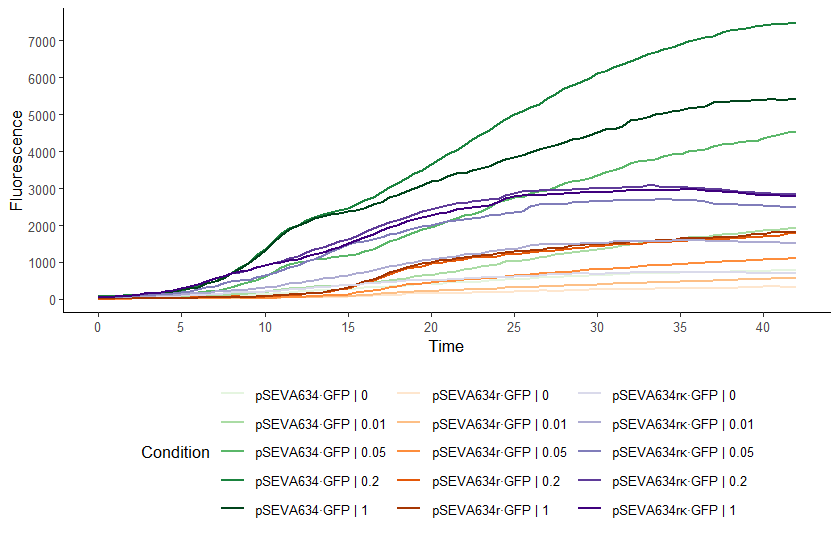Raw data plot. Conditions can be selected or deselected using the names = c('grp1', 'grp2') argument or exclude.nm = c('grp3', 'grp4') argument, respectively. Similarly, concentrations can be (de-selected) via the conc and exclude.conc arguments. To plot individual samples instead of grouping replicates, add mean = FALSE. See ?plot.grodata for further options.

## Run Workflow

flFitRes <- fl.workflow(grodata = input,
fit.opt = c("s", "l"),
x_type = "time",
norm_fl = TRUE,
ec50 = TRUE,
dr.parameter = "dY.spline",
suppress.messages = TRUE,
export.res = FALSE, # Prevent creating TXT table and RData files with results
parallelize = FALSE) # Use only one available CPU core

If option export.res is set to TRUE, tab-delimited .txt files summarizing the computation results are created automatically, as well as the flFitRes object (an object of class flFitRes) as .RData file. This object (or the .RData file) contains all raw data, fitting options, and computational results. Figure @ref(fig:flFitRes-container) shows the structure of the generated flFitRes object. In RStudio, View(flFitRes) allows interactive inspection of the data container.

If you want to create a report summarizing all computational results including a graphical representation of every fit, provide the desired output format(s) as report = 'pdf', report = 'html', or report = c('pdf', 'html'). The advantage of having the report in HTML format is that every figure can be exported as (editable) PDF file.

In the spirit of good scientific practice (data transparency), I would encourage anyone using QurvE to attach the .RData file and generated reports to their publication.

Arguments that are commonly modified:

 fit.opt Which growth fitting methods to perform; a string containing 'l' for linear fits or 's' for spline fits. Both fit types can be selected as a vector of strings: c('l', 's'). x_type Data type used as independent variable. Either 'growth' or 'time'. norm_fl Use normalized fluorescence values for curve fitting (if x_type = 'time'). log.y.lin log.y.spline Should Ln(y/y0) be applied to the fluorescence data for the respective fits? biphasic Extract parameters for two different phases (as observed with, e.g., diauxic shifts) interactive Controls interactive mode. If TRUE, each fit is visualized in the Plots pane and the user can adjust fitting parameters and confirm the reliability of each fit per sample nboot.fl Number of bootstrap samples used for nonparametric curve fitting. See ?flBootSpline for details. dr.method Define the method used to perform a dose-responde analysis: smooth spline fit ('spline') or model fitting ('model', the default). See section 4 dr.parameter The response parameter in the output table to be used for creating a dose response curve. See ?fl.drFit for further details.

Please consult ?fl.workflow for further arguments to customize the workflow.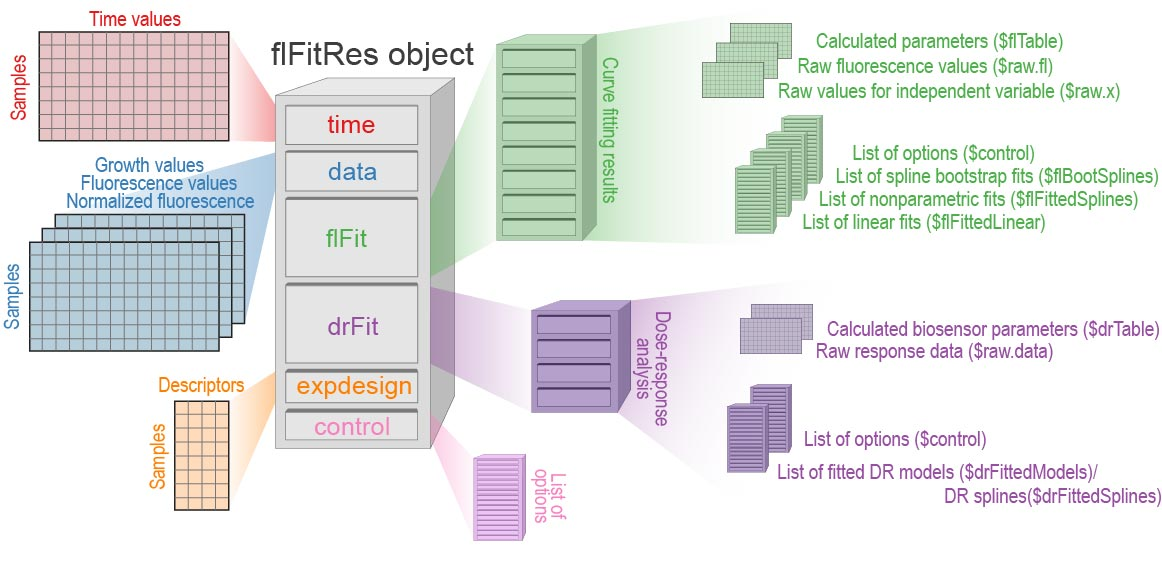Internal structure of a flFitResobject generated by growth.workflow().

## Tabular results

A flFitRes object contains two tables summarizing the computational results: - flFitRes$flFit$flable lists all calculated curve parameters for every sample and fit - flFitRes$drFit$drTable contains the results of the dose-response analysis

Additionally, the dedicated functions table_group_fluorescence_linear() and table_group_fluorescence_spline() allow the generation of grouped results tables for the two fit types with averages and standard deviations. The column headers in the resulting data frames are formatted with HTML for visualization in shiny and with DT::datatable().

A summary of results for each individual fit can be obtained by applying the generic function summary() to any fit object within flFitRes.

## Visualize results

Several generic plot() allow plotting of results by merely accessing list items within the flFitRes object structure (Figure @ref(fig:flFitRes-container)).

### Inspect individual fits

It is important to verify the accuracy of the fits that have been applied before attempting to interpret any results (if the workflow is not run with interactive = TRUE. This is especially important when analyzing fluorescence data, as the curve shapes and the level of noise can vary significantly depending on the specific experiment and the equipment used for cultivation.

plot(flFitRes$flFit$flFittedLinear[], cex.lab = 1.2, cex.axis = 1.2)
plot(flFitRes$flFit$flFittedLinear[], cex.lab = 1.2, cex.axis = 1.2)
plot(flFitRes$flFit$flFittedLinear[], cex.lab = 1.2, cex.axis = 1.2)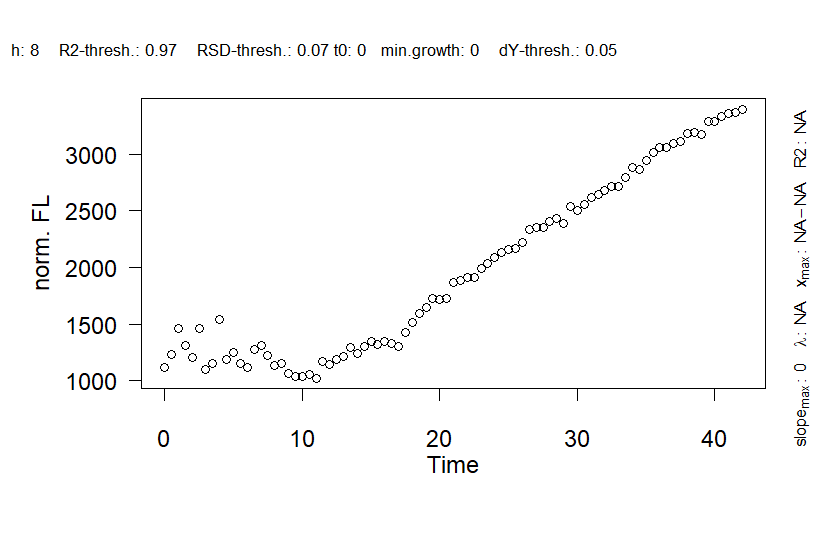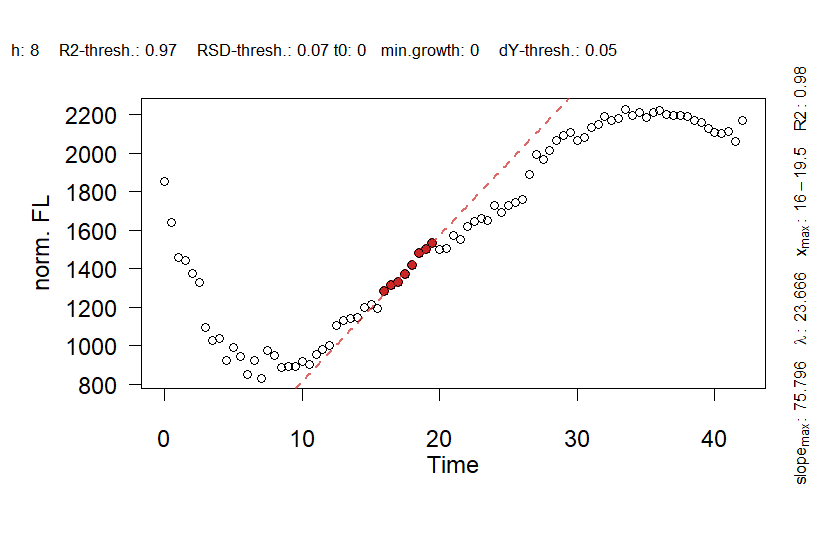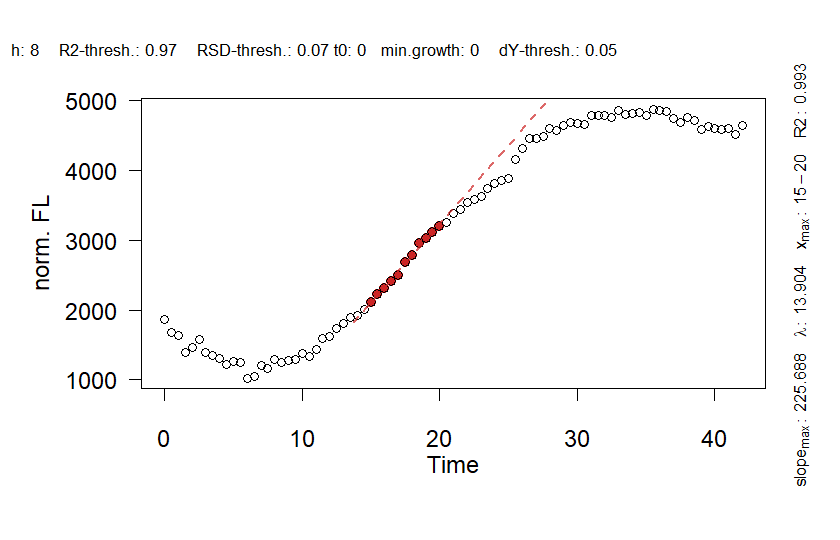Linear fit plots to validate the applied fits. For details about this function, run ?plot.gcFitLinear.

plot(flFitRes$flFit$flFittedSpline[], basesize = 15)
plot(flFitRes$flFit$flFittedSpline[], basesize = 15)
plot(flFitRes$flFit$flFittedSpline[], basesize = 15)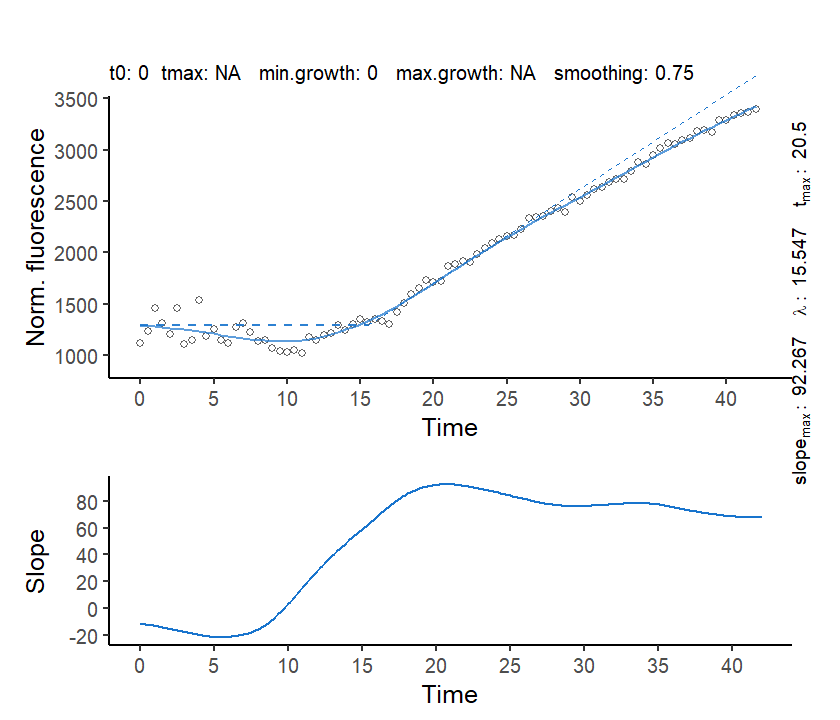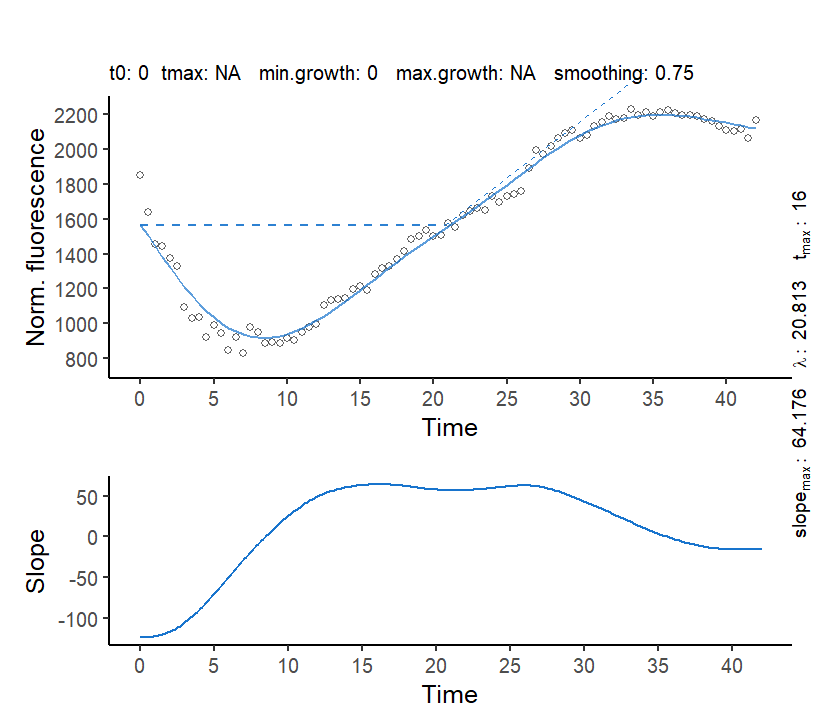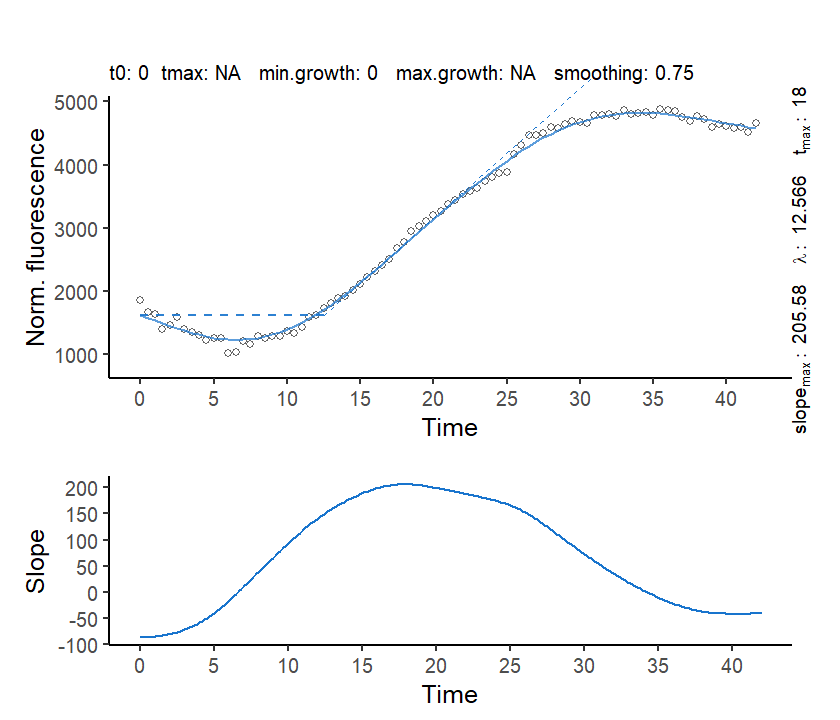Spline fit plots to validate the applied fits. For details about this function, run ?plot.gcFitLinear.

Normalization of fluorescence reads typically introduces additional noise. While the default smoothing parameter smooth.fl was suitable to produce good-quality representations of the curves via nonparametric fits, the linear fits either failed or produced regression windows that were too small. In order to obtain linear regression results that accurately represent the linear-increase section of the curves, we have to decrease the R2 threshold and manually increase the size of the sliding window (by default calculated automatically for each sample). These new settings need to be applied to all samples, so we re-run the entire workflow with adjusted parameters:

flFitRes <- fl.workflow(grodata = input,
fit.opt = c("s", "l"),
x_type = "time",
norm_fl = TRUE,
lin.R2 = 0.95, # Decreased R2 threshold
lin.h = 20, # Manually defined sliding window size
ec50 = TRUE,
dr.parameter = "dY.spline",
suppress.messages = TRUE,
export.res = FALSE,
parallelize = FALSE)
plot(flFitRes$flFit$flFittedLinear[], cex.lab = 1.2, cex.axis = 1.2)
plot(flFitRes$flFit$flFittedLinear[], cex.lab = 1.2, cex.axis = 1.2)
plot(flFitRes$flFit$flFittedLinear[], cex.lab = 1.2, cex.axis = 1.2)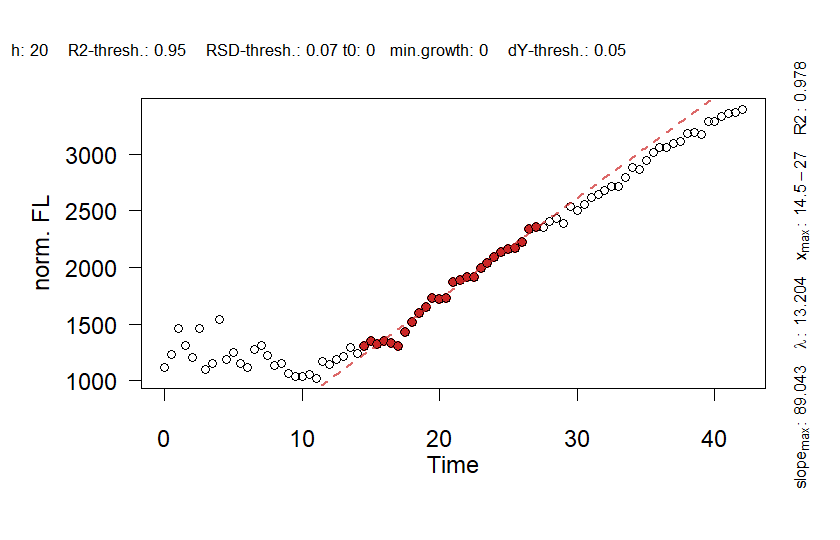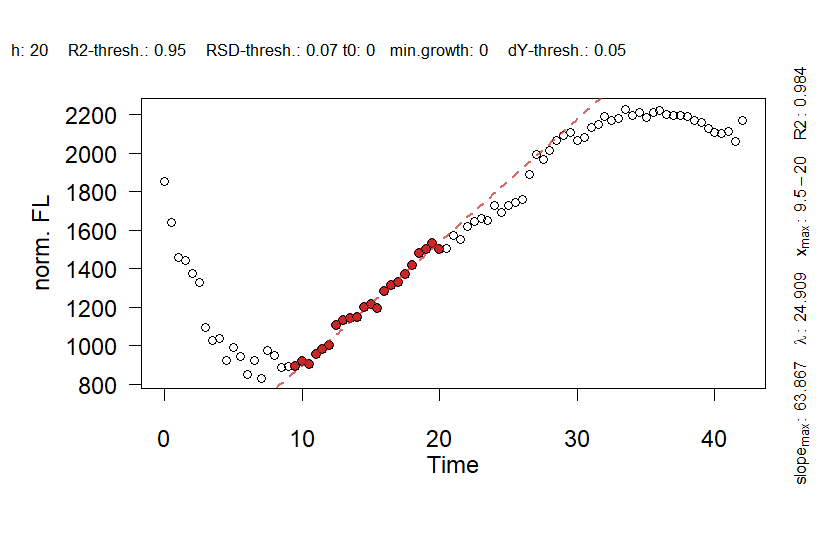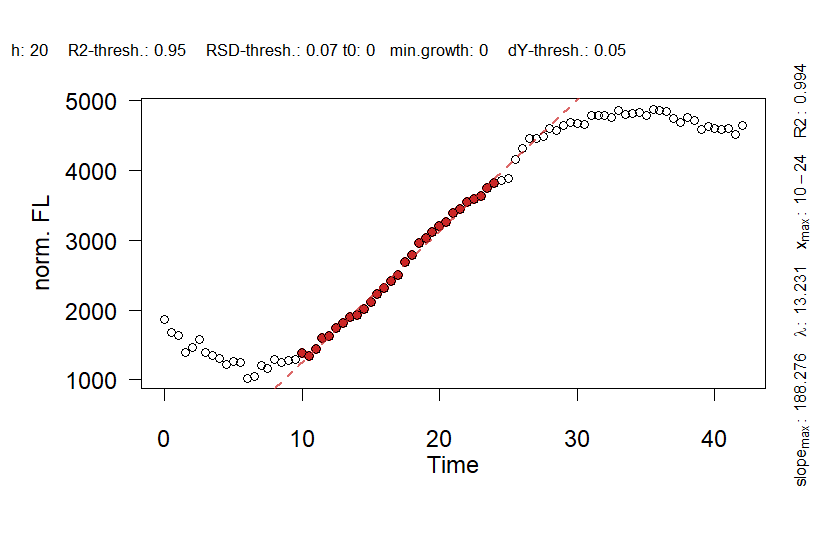Linear fit plots to validate the linear regressions after re-running the workflow with adjusted parameters.

### Grouped spline fits

Applying plot() to the flFitRes object produces a figure of all spline fits performed as well as the first derivative (slope) over time. The generic function calls plot.flFitRes() with data.type = 'spline'.

plot(flFitRes,
data.type = "spline",
deriv = TRUE,
legend.position = "bottom",
legend.ncol = 3,
n.ybreaks = 10,
basesize=10,
lwd = 0.7)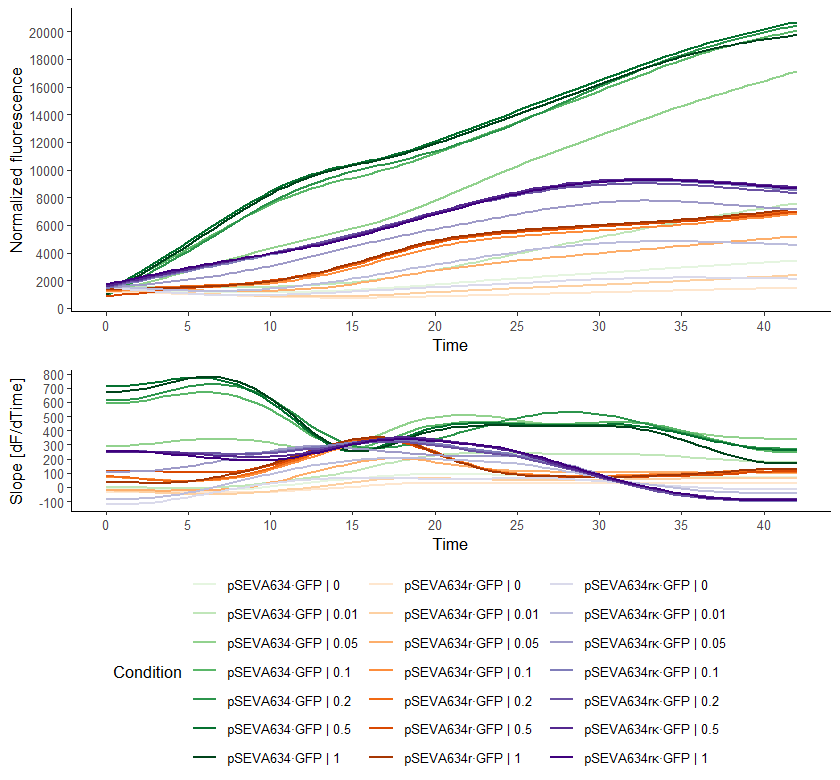Combined plot of all spline fits performed. In addition to the options available with data.type = 'raw', further arguments can be defined that control the appearance of the secondary panel showing the slope over time. See ?plot.flFitRes for all options.

By arranging the individual samples in a grid, we can create a visual representation similar to a heat map that illustrates the values of a chosen parameter. This can be a helpful way to gain insights and understand trends within the data.:

plot.grid(flFitRes,
param = "max_slope.spline",
pal = "Mint",
log.y = FALSE,
basesize = 12)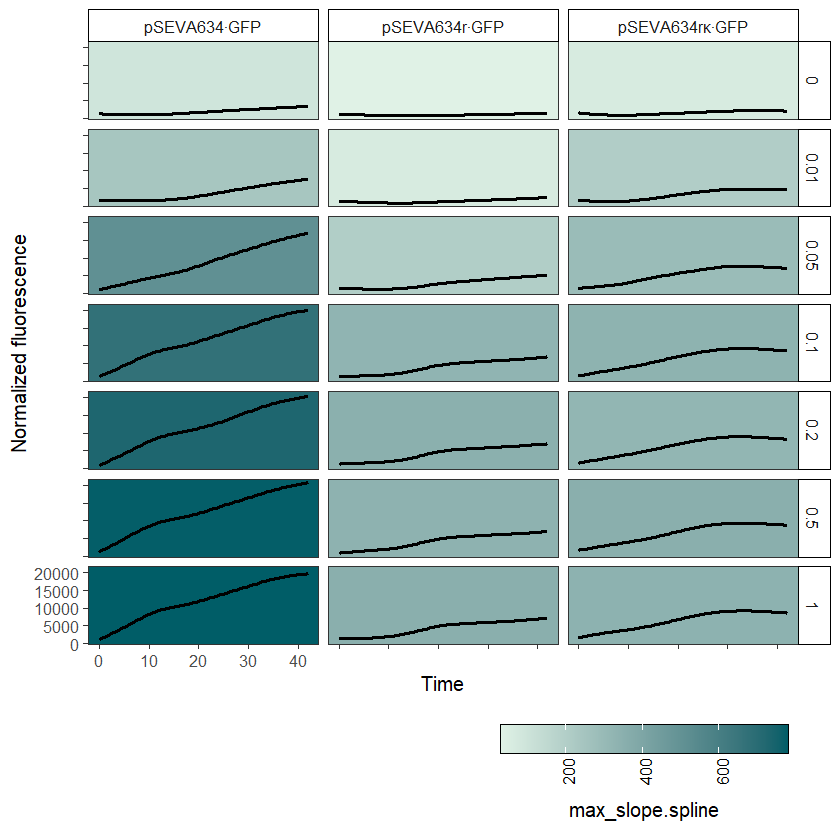Plot grid of all spline fits performed with one concentration per row. See ?plot.grid for available options.

### Compare growth parameters

The function plot.parameter() works also on flFitRes objects to compare computed curve parameters:

# Parameters obtained from linear regression
plot.parameter(flFitRes, param = "max_slope.linfit", basesize = 10,
legend.position = "bottom")
plot.parameter(flFitRes, param = "dY.linfit", basesize = 10,
legend.position = "bottom")

# Parameters obtained from nonparametric fits
plot.parameter(flFitRes, param = "max_slope.spline", basesize = 10,
legend.position = "bottom")
plot.parameter(flFitRes, param = "dY.spline", basesize = 10,
legend.position = "bottom")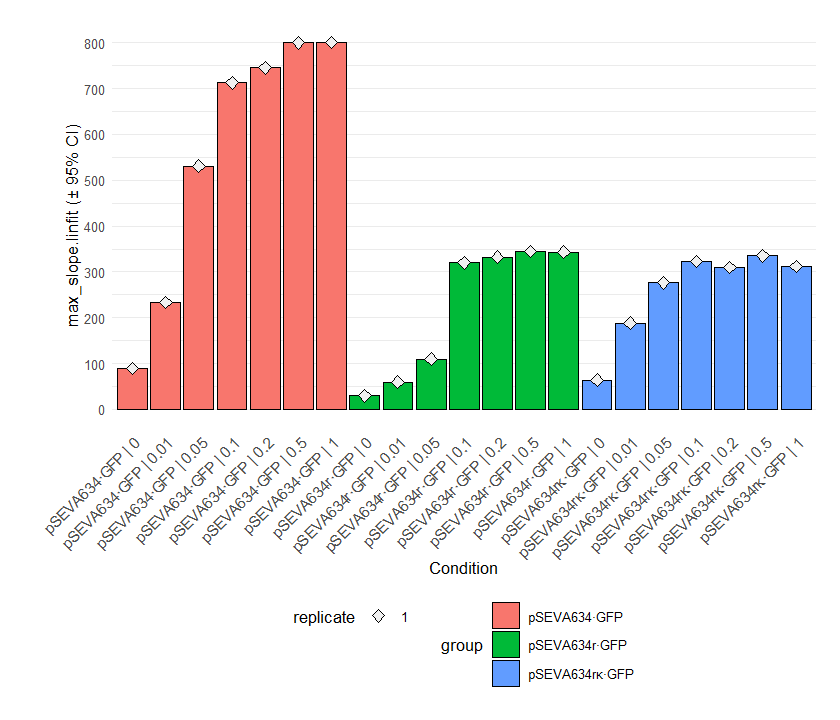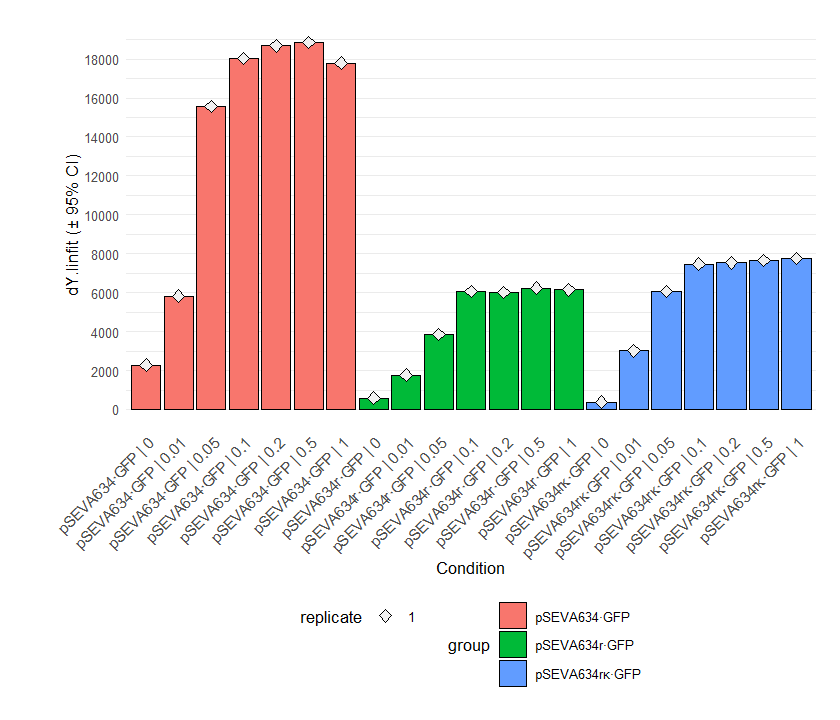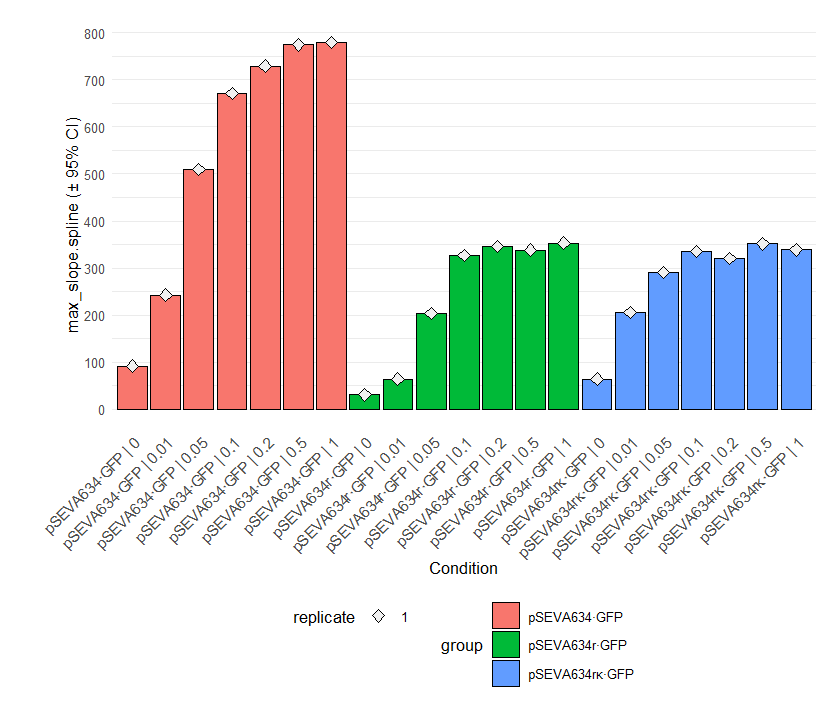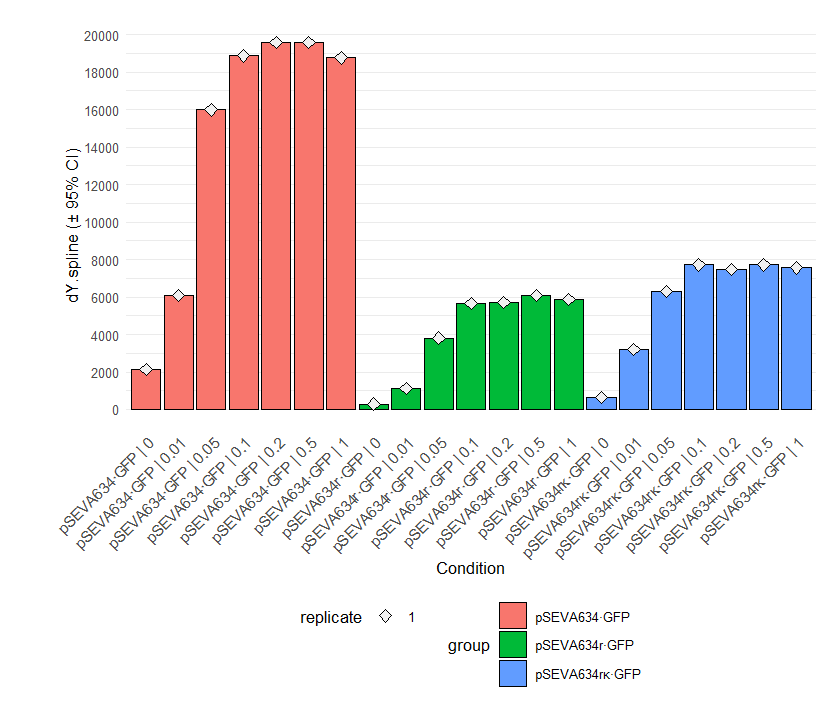Parameter plots. If mean = TRUE, the results of replicates are combined and shown as their mean ± 95% confidence interval. As with the functions for combining different growth curves, the arguments name, exclude.nm, conc and exclude.conc allow (de)selection of specific samples or conditions. Since we applied growth models to log-transformed data, calling ‘dY.orig.model’ or ‘A.orig.model’ instead of ‘dY.model’ or ‘A.model’ provides the respective values on the original scale. For linear and spline fits, this is done automatically. For details about this function, run ?plot.parameter.

### Dose-response analysis

The results of the dose-response analysis can be visualized by calling plot() on the drFit object that is stored within flFitRes. This action calls plot.drFit() which in turn runs plot.drFitSpline() or plot.drFitModel() (depending on the choice of in the workflow) on every condition for which a dose-response analysis has been performed. Alternatively, you can call plot() on the list elements in grofit$drFit$drFittedModels or grofit$drFit$drFittedSplines, respectively.

plot(flFitRes\$drFit, cex.point = 1, cex.lab = 1.1, cex.axis = 1)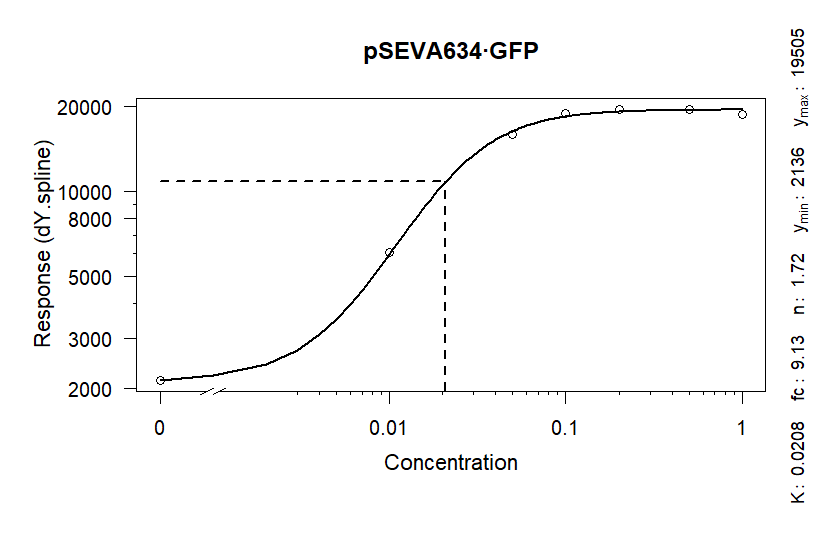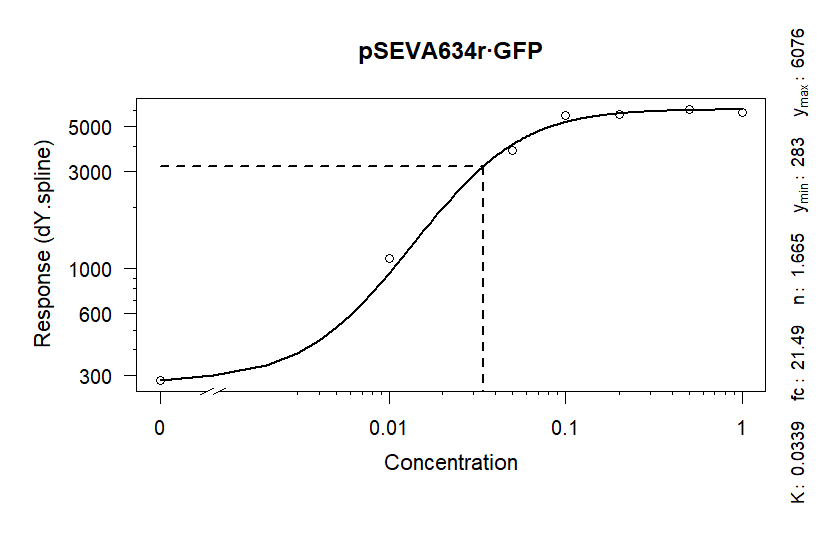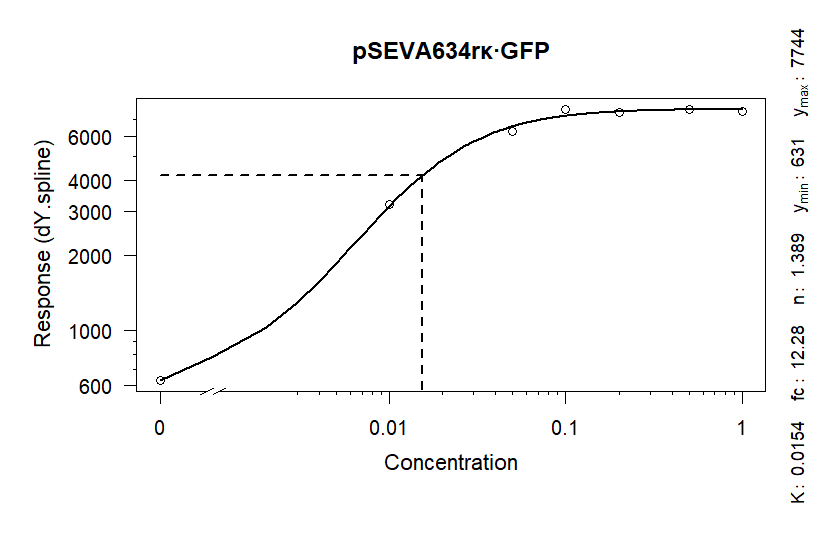Dose response analysis - model fits. For details about this function, run ?plot.drFit.